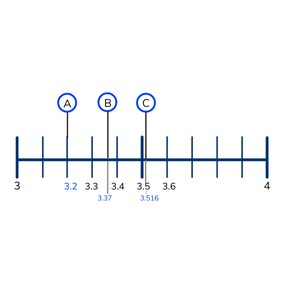Place value- decimal numbers with 1, 2, or 3 decimal places on the number line

# Place value- decimal numbers with 1, 2, or 3 decimal places on the number line

Place value- decimal numbers with 1, 2, or 3 decimal places on the number line

No account needed.8,000 schools use Gynzy92,000 teachers use Gynzy1,600,000 students use Gynzy

## General

Students learn to place decimal numbers with different decimal places (1,2, and 3) on the number line.

## Common core standard(s)

CCSS.Math.Content.5.NBT.A.3

## Relevance

It is important to be able to place decimal numbers on the number line to be able to compare them. If you are able to place a decimal number on the number line you can tell which numbers are less than or greater than your number.

## Introduction

Start with linking decimal numbers to objects. Ask students how they know to match the decimal number to the objects. Amounts are always written with 2 decimal places, for example. Next complete the number sequences. The first increases by 0.1, the second by 0.01, and the third by 0.001.

## Instruction

Tell students that they should always start by looking at the starting number and final number on a number line, so you know the range of a number line. Then you count the number of intermediate marks, so you know how much each mark represents- sometimes they are tenths, hundredths or thousandths. When you know what the marks represent, you are able to place your number. Practice this on different number lines. One with 10 marks, one with 100, marks, with 5 marks and with 50 marks. Repeat the steps every time you place a new number. Then show a number line that has two numbers marked, one with tenths, and one with hundredths. The tenths are on the long marks, the hundredths on the short marks. To determine the tenths count from 9.0 to 9.1, 9.02, 9.3. To determine the hundredths, first determine the neighboring tenths numbers and then count from 9.7 to 9.71, 9.72. Check that students understand by placing similar numbers on similar number lines. Always start by checking the starting number and final number, then determine neighboring tenths numbers, and placing your number.

Check that students understand placing decimal numbers with 1,2, or 3 decimal places on the number line by asking the following questions:
- What are the neighboring hundredths of 0.034?
- Which number is exactly in the middle between 8.6 and 8.7?
- Which number is exactly in the middle between 8.61 and 8.62?

## Guided practice

Practice placing decimal numbers with 1, 2, or 3 decimal places on the number line together. First on a number line from 4 to 5, then from 0 to 0.5 and then from 4.5 to 5 without intermediate marks.

## Closing

Discuss the steps of placing a number on the number line with your students. First always look at what the starting number and final number are. Then you count the marks to determine what amount the represent, or you place imaginary marks. Then determine the neighboring tenths or hundredths and then place your number. Check with a final exercise that students understand placing decimal numbers with 1, 2, or 3 decimals on the number line using a number line from 7.2 to 7.3. It is useful to write the hundredths on the number line. Ask students to explain their thinking.

## Teaching tips

Students who are not as comfortable with the number line can be confused by the changes of starting and final numbers, as well as the changes in value of intermediate marks. Take time to review the steps with the students and emphasize the importance of determining the starting and final numbers of the number line, and taking the time to determine the value of the intermediate marks. You can also have them practice on a number line to 100, telling them that the only difference is that a decimal point is in the number.

### The online teaching platform for interactive whiteboards and displays in schools

• Save time building lessons

• Manage the classroom more efficiently

• Increase student engagement